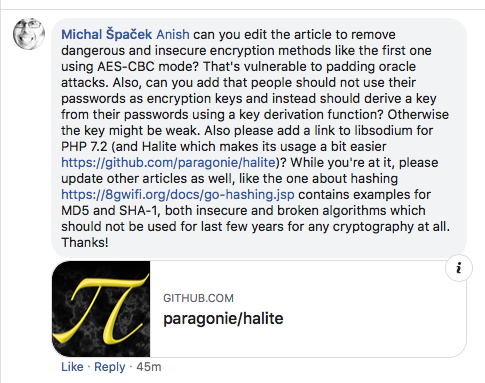# php Encryption/Decryption

by Anish

Posted on Thursday December 13 , 2018

## openssl_encrypt/decrypt

openssl_encrypt: Encrypt the data
openssl_decrypt: Decrypt the data

• Supported PHP Versions (PHP 5 >= 5.3.0, PHP 7)

The Syntax

``````string openssl_encrypt ( string \$data , string \$method , string \$key [, int \$options = 0 [, string \$iv = "" [, string \$tag = NULL [, string \$aad = "" [, int \$tag_length = 16 ]]]]] )
``````
``````string openssl_decrypt ( string \$data , string \$method , string \$key [, int \$options = 0 [, string \$iv = "" [, string \$tag = "" [, string \$aad = "" ]]]] )
``````
• data: The plaintext message data to be encrypted.
• method: The cipher method, see openssl_get_cipher_methods() for a list of potential values.
• key : User supplied key
• IV: The Initialization Vector
• Returns: Returns the encrypted string on success or `FALSE` on failure.

Since block cipher modes of operation require an IV which is random and unpredictable, or at least unique for each message encrypted with a given key. Different block cipher modes have different IV length requirement for example

Cipher Mode IV Length
aes-128-cbc 16
camellia-192-ofb 16
cast5-cbc 8
bf-cbc 8
aes-256-gcm 12

Examples

A Great Feedback by Michal Špaček### aes-128-cbc encrypt/decrypt example

Note: AES-CBC mode vulnerable to padding oracle attacks, Kindly Don't use these

``````/**
* Created by https://8gwifi.org
* User: Anish Nath
* Date: 2018-12-10
* Time: 15:13
*/

<?php

// php AES-128-CBC Encryption Example
\$plaintext = "Hello 8gwifi.org";

\$ivlen = openssl_cipher_iv_length(\$cipher="aes-128-cbc");

//Generate Random IV
\$iv = openssl_random_pseudo_bytes(\$ivlen);
\$ciphertext_raw = openssl_encrypt(\$plaintext, \$cipher, \$key, \$options=OPENSSL_RAW_DATA, \$iv);
\$ciphertext = base64_encode( \$iv.\$ciphertext_raw );

echo "Encrypted Text : \$ciphertext \n";

//php AES-128-CBC Dec Example
\$c = base64_decode("\$ciphertext");
\$ivlen = openssl_cipher_iv_length(\$cipher="aes-128-cbc");
\$iv = substr(\$c, 0, \$ivlen);
\$ciphertext_raw = substr(\$c, \$ivlen);

\$original_plaintext = openssl_decrypt(\$ciphertext_raw, \$cipher, \$key, \$options=OPENSSL_RAW_DATA, \$iv);
echo "Decrypted Text: \$original_plaintext\n";

?>
``````

The above example will output something similar to:

``````\$ /usr/bin/php aes-128-cbc.php
/**
* Created by https://8gwifi.org
* User: Anish Nath
* Date: 2018-12-10
* Time: 15:13
*/
Encrypted Text : CLBckQqECD15NoXSD3JZGknFlQtpGrYR7BFlUshUZ9t7pMdAz6FAR+dkym46+stX
Decrypted Text: Hello 8gwifi.org

Process finished with exit code 0
``````

### aes-192-cfb encrypt/decrypt example

``````/**
* Created by https://8gwifi.org
* User: Anish Nath
* Date: 2018-12-10
* Time: 15:13
*/

<?php

// php AES-192-CBC Encryption Example
\$plaintext = "Hello 8gwifi.org";

\$ivlen = openssl_cipher_iv_length(\$cipher="aes-192-cfb");
//Generate Random IV
\$iv = openssl_random_pseudo_bytes(\$ivlen);
\$ciphertext_raw = openssl_encrypt(\$plaintext, \$cipher, \$key, \$options=OPENSSL_RAW_DATA, \$iv);

\$ciphertext = base64_encode( \$iv.\$ciphertext_raw );
echo "Encrypted Text : \$ciphertext \n";

//php AES-192-CBC Dec Example
\$c = base64_decode(\$ciphertext);
\$ivlen = openssl_cipher_iv_length(\$cipher="aes-192-cfb");
\$iv = substr(\$c, 0, \$ivlen);
\$ciphertext_raw = substr(\$c, \$ivlen);
\$original_plaintext = openssl_decrypt(\$ciphertext_raw, \$cipher, \$key, \$options=OPENSSL_RAW_DATA, \$iv);

echo "Decrypted Text: \$original_plaintext\n";

?>
``````

The above example will output something similar to:

``````\$ php aes-192-cfb.php
Encrypted Text : /72Gdi7jAqKVJ7APgtWXPKB+9s3+iS7/vwgOaO5/LEc=
Decrypted Text: Hello 8gwifi.org
``````

### aes-256-ofb encrypt/decrypt example

``````
<?php
// php AES-256-OFB Dec Example
\$plaintext = "Hello 8gwifi.org";

\$ivlen = openssl_cipher_iv_length(\$cipher="aes-256-ofb");
//Generate Random IV
\$iv = openssl_random_pseudo_bytes(\$ivlen);
\$ciphertext_raw = openssl_encrypt(\$plaintext, \$cipher, \$key, \$options=OPENSSL_RAW_DATA, \$iv);

\$ciphertext = base64_encode( \$iv.\$ciphertext_raw );

echo "Encrypted Text : \$ciphertext \n";

//php AES-256-OFB Dec Example
\$c = base64_decode(\$ciphertext);
\$ivlen = openssl_cipher_iv_length(\$cipher="aes-256-ofb");
\$iv = substr(\$c, 0, \$ivlen);
\$ciphertext_raw = substr(\$c, \$ivlen);
\$original_plaintext = openssl_decrypt(\$ciphertext_raw, \$cipher, \$key, \$options=OPENSSL_RAW_DATA, \$iv);

echo "Decrypted Text: \$original_plaintext\n";

?>
``````

The above example will output something similar to:

``````\$/usr/bin/php aes-256-ofb.php
Encrypted Text : FtsxVyVc5zi/x/1oEpEoaVzNJKw6bYkgDH2kST2PLug=
Decrypted Text: Hello 8gwifi.org
``````

### aes-256-gcm encrypt/decrypt example

Note: AEAD is not supported for all version prior to the PHP 7.1, This example utilizes php 7.1

``````/**
* Created by https://8gwifi.org
* User: Anish Nath
* Date: 2018-12-10
* Time: 15:13
*/

<?php

// php aes-256-gcm Encryption Example
\$plaintext = "Hello 8gwifi.org";

\$ivlen = openssl_cipher_iv_length(\$cipher="aes-256-gcm");

//Generate Random IV
\$iv = openssl_random_pseudo_bytes(\$ivlen);

\$ciphertext_raw = openssl_encrypt(\$plaintext, \$cipher, \$key, \$options=OPENSSL_RAW_DATA, \$iv,\$aad);

\$ciphertext = base64_encode( \$iv.\$ciphertext_raw );

echo "Encrypted Text : \$ciphertext \n";

//php aes-256-gcm Dec Example
\$c = base64_decode(\$ciphertext);
\$ivlen = openssl_cipher_iv_length(\$cipher="aes-256-gcm");
\$iv = substr(\$c, 0, \$ivlen);
\$ciphertext_raw = substr(\$c, \$ivlen);
\$original_plaintext = openssl_decrypt(\$ciphertext_raw, \$cipher, \$key, \$options=OPENSSL_RAW_DATA, \$iv,\$aad);
echo "Decrypted Text: \$original_plaintext\n";
?>
``````

The above example will output something similar to:

``````\$ /bin/php-7.1.19 aes-256-gcm.php
/**
* Created by https://8gwifi.org
* User: Anish Nath
* Date: 2018-12-10
* Time: 15:13
*/
Encrypted Text : p3pzMb42n03a9GZTwLHHVf2EPT8OcKrbAg/CVQ==
Decrypted Text: Hello 8gwifi.org
``````

### camellia-256-ofb encrypt/decrypt example

``````/**
* Created by https://8gwifi.org
* User: Anish Nath
* Date: 2018-12-10
* Time: 15:13
*/

<?php

// php camellia-256-ofb Encryption Example
\$plaintext = "Hello 8gwifi.org";
\$ivlen = openssl_cipher_iv_length(\$cipher="camellia-256-ofb");

//Generate Random IV
\$iv = openssl_random_pseudo_bytes(\$ivlen);

\$ciphertext_raw = openssl_encrypt(\$plaintext, \$cipher, \$key, \$options=OPENSSL_RAW_DATA, \$iv);

\$ciphertext = base64_encode( \$iv.\$ciphertext_raw );

echo "Encrypted Text : \$ciphertext \n";

//php camellia-256-ofb Dec Example
\$c = base64_decode(\$ciphertext);
\$ivlen = openssl_cipher_iv_length(\$cipher="camellia-256-ofb");
\$iv = substr(\$c, 0, \$ivlen);
\$ciphertext_raw = substr(\$c, \$ivlen);
\$original_plaintext = openssl_decrypt(\$ciphertext_raw, \$cipher, \$key, \$options=OPENSSL_RAW_DATA, \$iv);

echo "Decrypted Text: \$original_plaintext\n";

?>
``````

The above example will output something similar to:

``````/usr/bin/php camellia-256-ofb.php
Encrypted Text : AgSQP79ml7W/sTBWdtmzkQRgMc8R2L2Nm3LnrOC2+xc=
Decrypted Text: Hello 8gwifi.org
``````

Thanku for reading !!! Give a Share for Support

Asking for donation sound bad to me, so i'm raising fund from by offering all my eight book for just \$9##### Online Terminals